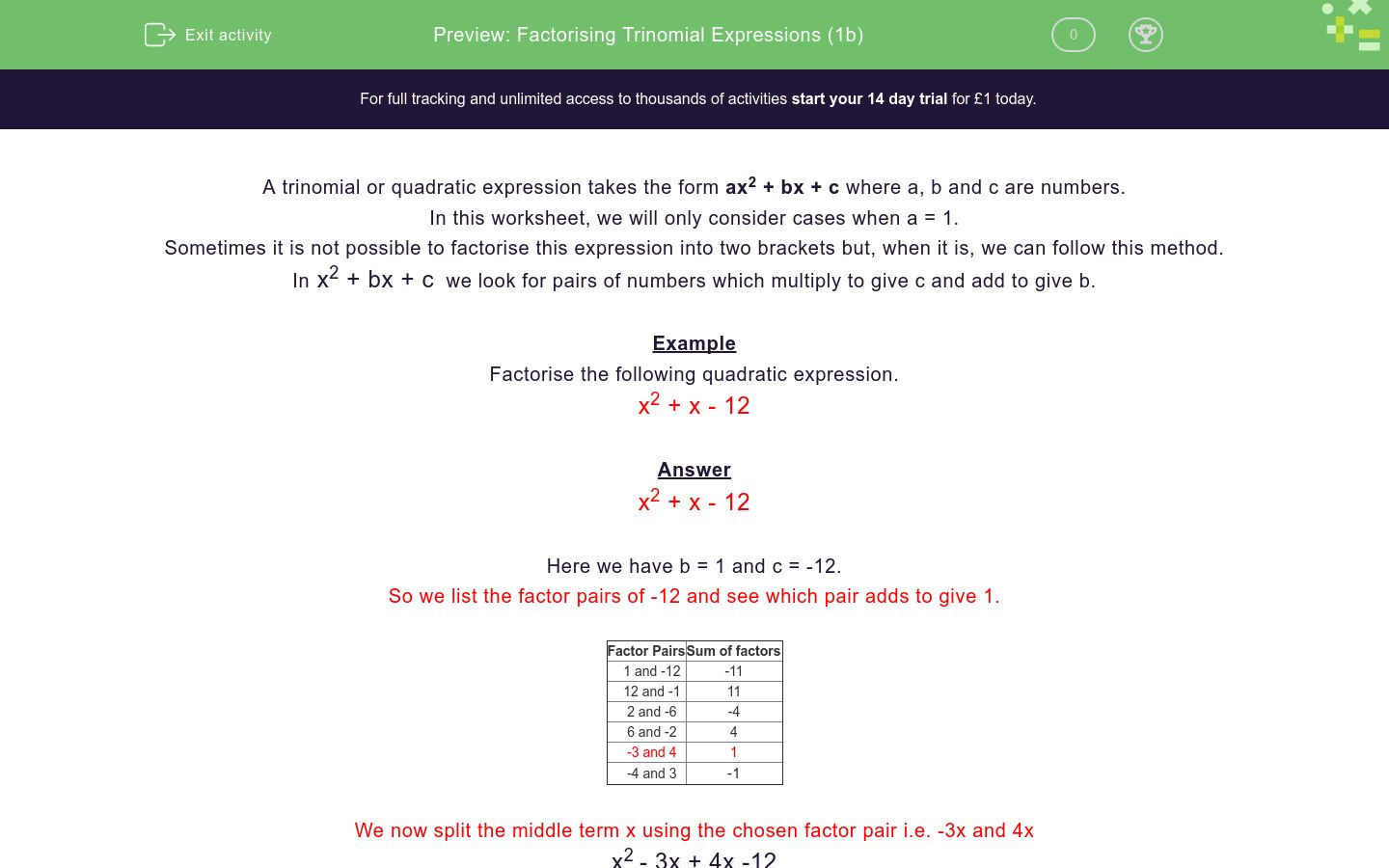# Factorising Trinomial Expressions (1b)

In this worksheet, students factorise simple trinomial expressions.Key stage:  KS 4

Curriculum topic:  Algebra

Curriculum subtopic:  Simplify/Manipulate Algebraic Expressions/Fractions

Difficulty level:### QUESTION 1 of 10

A trinomial or quadratic expression takes the form ax2 + bx + c where a, b and c are numbers.

In this worksheet, we will only consider cases when a = 1.

Sometimes it is not possible to factorise this expression into two brackets but, when it is, we can follow this method.

In x2 + bx + c  we look for pairs of numbers which multiply to give c and add to give b.

Example

Factorise the following quadratic expression.

x2 + x - 12

Answer

x2 + x - 12

Here we have b = 1 and c = -12.

So we list the factor pairs of -12 and see which pair adds to give 1.

Factor Pairs Sum of factors
1 and -12 -11
12 and -1 11
2 and -6 -4
6 and -2 4
-3 and 4 1
-4 and 3

-1

We now split the middle term x using the chosen factor pair i.e. -3x and 4x

x2 - 3x + 4x -12

Consider the expression in pairs

x2 - 3x + 4x - 12

Factorise each pair

x (x - 3) + 4 (x - 3)

Combine

(x + 4) (x - 3)

This is the full factorisation

(x + 4) (x - 3)

Factorise the following quadratic expression.

x2 + 5x - 6

(x + 2) (x - 4)

(x - 3) (x + 2)

(x + 6) (x - 1)

(x + 2) (x + 6)

Factorise the following quadratic expression.

x2 + x - 6

(x - 2) (x + 6)

(x - 1) (x + 6)

(x + 3) (x - 2)

(x + 2) (x - 4)

Factorise the following quadratic expression.

x2 + 2x - 8

(x + 2) (x - 6)

(x - 1) (x + 8)

(x + 3) (x - 2)

(x - 2) (x + 4)

Factorise the following quadratic expression.

x2 - 2x - 8

(x - 2) (x - 4)

(x - 1) (x + 8)

(x - 3) (x + 2)

(x + 2) (x - 4)

Factorise the following quadratic expression.

x2 - 8x - 9

(x + 3) (x - 3)

(x + 1) (x - 9)

(x - 2) (x + 8)

(x + 4) (x - 4)

Factorise the following quadratic expression.

x2 + 8x - 9

(x - 3) (x + 3)

(x - 1) (x + 9)

(x + 2) (x - 8)

(x - 4) (x + 4)

Factorise the following quadratic expression.

x2 + 6x - 16

(x + 3) (x - 3)

(x + 1) (x - 9)

(x - 2) (x + 8)

(x + 4) (x - 4)

Factorise the following quadratic expression.

x2 + 0x - 9

(x + 3) (x - 3)

(x + 1) (x - 9)

(x - 2) (x + 8)

(x + 4) (x - 4)

Factorise the following quadratic expression.

x2 + 16x - 36

(x + 18) (x - 2)

(x - 12) (x + 3)

(x + 6) (x - 6)

(x + 9) (x - 4)

Factorise the following quadratic expression.

x2 - 9x - 36

(x + 18) (x - 2)

(x - 12) (x + 3)

(x + 6) (x - 6)

(x - 9) (x - 4)

• Question 1

Factorise the following quadratic expression.

x2 + 5x - 6

CORRECT ANSWER
(x + 6) (x - 1)
• Question 2

Factorise the following quadratic expression.

x2 + x - 6

CORRECT ANSWER
(x + 3) (x - 2)
• Question 3

Factorise the following quadratic expression.

x2 + 2x - 8

CORRECT ANSWER
(x - 2) (x + 4)
• Question 4

Factorise the following quadratic expression.

x2 - 2x - 8

CORRECT ANSWER
(x + 2) (x - 4)
• Question 5

Factorise the following quadratic expression.

x2 - 8x - 9

CORRECT ANSWER
(x + 1) (x - 9)
• Question 6

Factorise the following quadratic expression.

x2 + 8x - 9

CORRECT ANSWER
(x - 1) (x + 9)
• Question 7

Factorise the following quadratic expression.

x2 + 6x - 16

CORRECT ANSWER
(x - 2) (x + 8)
• Question 8

Factorise the following quadratic expression.

x2 + 0x - 9

CORRECT ANSWER
(x + 3) (x - 3)
EDDIE SAYS
This is really x2 - 9
• Question 9

Factorise the following quadratic expression.

x2 + 16x - 36

CORRECT ANSWER
(x + 18) (x - 2)
• Question 10

Factorise the following quadratic expression.

x2 - 9x - 36

CORRECT ANSWER
(x - 12) (x + 3)
---- OR ----

Sign up for a £1 trial so you can track and measure your child's progress on this activity.

### What is EdPlace?

We're your National Curriculum aligned online education content provider helping each child succeed in English, maths and science from year 1 to GCSE. With an EdPlace account you’ll be able to track and measure progress, helping each child achieve their best. We build confidence and attainment by personalising each child’s learning at a level that suits them.

Start your £1 trial